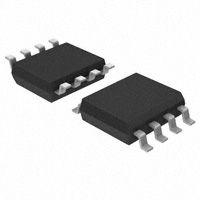< id="6e2kg">< id="6e2kg">< id="6e2kg">< id="6e2kg">< id="6e2kg">< id="6e2kg">< id="6e2kg">< id="6e2kg">< id="6e2kg">< id="6e2kg">< id="6e2kg">< id="6e2kg">< id="6e2kg">< id="6e2kg">< id="6e2kg">< id="6e2kg">< id="6e2kg">< id="6e2kg">< id="6e2kg">< id="6e2kg">< id="6e2kg">< id="6e2kg">< id="6e2kg">< id="6e2kg">< id="6e2kg">< id="6e2kg">< id="6e2kg">< id="6e2kg">< id="6e2kg">< id="6e2kg">< id="6e2kg">< id="6e2kg">< id="6e2kg">< id="6e2kg">< id="6e2kg">< id="6e2kg">< id="6e2kg">< id="6e2kg">< id="6e2kg">< id="6e2kg">< id="6e2kg">< id="6e2kg">< id="6e2kg">< id="6e2kg">< id="6e2kg">< id="6e2kg">< id="6e2kg">< id="6e2kg">< id="6e2kg">< id="6e2kg"> < id="6e2kg">< id="6e2kg">< id="6e2kg">< id="6e2kg">< id="6e2kg">< id="6e2kg">< id="6e2kg">< id="6e2kg">< id="6e2kg">< id="6e2kg">< id="6e2kg">< id="6e2kg">< id="6e2kg">< id="6e2kg">< id="6e2kg">< id="6e2kg">< id="6e2kg">< id="6e2kg">< id="6e2kg">< id="6e2kg">< id="6e2kg">< id="6e2kg">< id="6e2kg">< id="6e2kg">< id="6e2kg">< id="6e2kg">< id="6e2kg">< id="6e2kg">< id="6e2kg">< id="6e2kg">< id="6e2kg">< id="6e2kg">< id="6e2kg">< id="6e2kg">< id="6e2kg">< id="6e2kg">< id="6e2kg">< id="6e2kg">< id="6e2kg">< id="6e2kg">< id="6e2kg">< id="6e2kg">< id="6e2kg">< id="6e2kg">< id="6e2kg">< id="6e2kg">< id="6e2kg">< id="6e2kg">< id="6e2kg">< id="6e2kg"> < id="6e2kg">< id="6e2kg">< id="6e2kg">< id="6e2kg">< id="6e2kg">< id="6e2kg">< id="6e2kg">< id="6e2kg">< id="6e2kg">< id="6e2kg">< id="6e2kg">< id="6e2kg">< id="6e2kg">< id="6e2kg">< id="6e2kg">< id="6e2kg">< id="6e2kg">< id="6e2kg">< id="6e2kg">< id="6e2kg">< id="6e2kg">< id="6e2kg">< id="6e2kg">< id="6e2kg">< id="6e2kg">< id="6e2kg">< id="6e2kg">< id="6e2kg">< id="6e2kg">< id="6e2kg">< id="6e2kg">< id="6e2kg">< id="6e2kg">< id="6e2kg">< id="6e2kg">< id="6e2kg">< id="6e2kg">< id="6e2kg">< id="6e2kg">< id="6e2kg">< id="6e2kg">< id="6e2kg">< id="6e2kg">< id="6e2kg">< id="6e2kg">< id="6e2kg">< id="6e2kg">< id="6e2kg">< id="6e2kg">< id="6e2kg">型号： IR2104S 厂商：International Rectifier 分类： 集成电路 ， PMIC - MOSFET，电桥驱动器 - 外部开关 描述： IC driver high/low side 8-soic PDF： 预览 报错 收藏 赞

Datasheet下载地址

 本地下载 >> 厂商下载 >>

IR2104S的详细信息

Datasheets： 95 Integrated Circuits (ICs) PMIC - MOSFET, Bridge Drivers - External Switch - Tube Half Bridge Non-Inverting 680ns 210mA 1 2 600V 10 V ~ 20 V -40°C ~ 125°C Surface Mount 8-SOIC (0.154", 3.90mm Width) 8-SOIC N *IR2104S
Data Sheet No. PD60046-S
( )&(PbF)
S
IR2104
HALF-BRIDGE DRIVER
Features
Product Summary
Floating channel designed for bootstrap operation
?
V
600V max.
130 mA / 270 mA
10 - 20V
OFFSET
Fully operational to +600V
Tolerant to negative transient voltage
dV/dt immune
Gate drive supply range from 10 to 20V
Undervoltage lockout
3.3V, 5V and 15V input logic compatible
Cross-conduction prevention logic
High side output in phase with input
I +/-
O
?
?
V
OUT
?
t
(typ.)
680 & 150 ns
520 ns
on/off
?
?
?
Shut down input turns off both channels
Matched propagation delay for both channels
?
Packages
?
? Also available LEAD-FREE
Description
The IR2104(S) are high voltage, high speed power
MOSFET and IGBT drivers with dependent high and low
side referenced output channels. Proprietary HVIC and
latch immune CMOS technologies enable ruggedized
IR2104S
IR2104
monolithic construction. The logic input is compatible with standard CMOS or LSTTL output, down to 3.3V logic.
The output drivers feature a high pulse current buffer stage designed for minimum driver cross-conduction. The
floating channel can be used to drive an N-channel power MOSFET or IGBT in the high side configuration which
operates from 10 to 600 volts.
Typical Connection
up to 600V
VCC
VCC
IN
VB
HO
VS
IN
TO
SD
SD
COM
LO
(Refer to Lead Assignment for correct pin configuration) This/These diagram(s) show electrical
connections only. Please refer to our Application Notes and DesignTips for proper circuit board layout.
www.irf.com
1